Geometry

Area of a rhombus calculator

Area of a rhombus calculator - formulas and calculator for calculating rhombus area. Area of a rhombus - is a numerical characteristic characterizing the size of a plane bounded by four series-connected segments (sides) of the same length, in which the opposite sides are parallel in pairs, and the angle between any two adjacent sides is not equal to 90 degrees.

Various formulas are used to calculate the rhombus area, depending on the known input data.

Area - is a numerical characteristic characterizing the size of a plane bounded by a closed geometric figure.

Area is measured in units of measurement squared: km2, m2, cm2, mm2, etc.

1

Area of the rhombus on side and heighta - side
h - height

... preparation ...

2

Area of the rhombus with two diagonals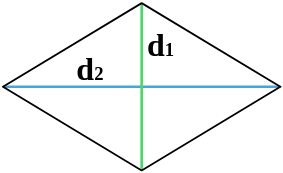d1 - diagonal
d2 - diagonal

... preparation ...

3

Area of the rhombus in the corner and the opposite diagonal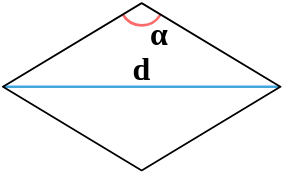d - diagonal
α° - angle between parties

... preparation ...

4

Area of the rhombus by angle and a diagonal drawn from that angle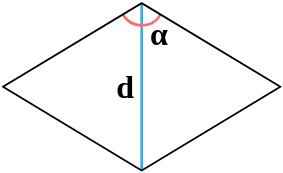d - diagonal
α° - angle between parties

... preparation ...

5

Area of the rhombus on the side and the corner between the sidesa - side
α° - angle between parties

... preparation ...

6

Area of the rhombus along the radius of the inscribed circle and the corner between the sides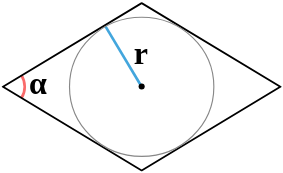r - radius of inscribed circle
α° - angle between parties

... preparation ...

7

Area of the rhombus on the radius of the inscribed circle and the side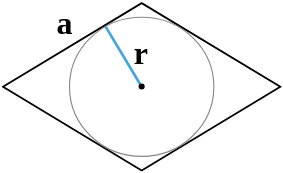a - side
r - radius of inscribed circle

... preparation ...